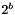# cu/ptr.h: Pointer Arithmetic [Data Types]

## Functions

void * cu_ptr_add (void *ptr, ptrdiff_t diff)
void * cu_ptr_sub (void *ptr, ptrdiff_t diff)
ptrdiff_t cu_ptr_diff (void const *ptr_lhs, void const *ptr_rhs)
int cu_ptr_cmp (void const *ptr0, void const *ptr1)
void * cu_ptr_mulfloor (void *ptr, cu_offset_t m)
void * cu_ptr_mulceil (void *ptr, cu_offset_t m)
void * cu_ptr_scal2floor (void *ptr, unsigned int b)
void * cu_ptr_scal2ceil (void *ptr, unsigned int b)
void * cu_ptr_alignfloor (void *ptr)
void * cu_ptr_alignceil (void *ptr)

## Function Documentation

 void* cu_ptr_add ( void * ptr, ptrdiff_t diff )

Add diff bytes to ptr irrespective of the underlying type.

 void* cu_ptr_alignceil ( void * ptr )

The pointer ptr rounded up to the nearest multiple of the maximum alignment.

 void* cu_ptr_alignfloor ( void * ptr )

The pointer ptr rounded down to the nearest multiple of the maximum alignment.

 int cu_ptr_cmp ( void const * ptr0, void const * ptr1 )

Return -1 if ptr0 < ptr1, 0 if ptr0 = ptr1, and 1 otherwise.

 ptrdiff_t cu_ptr_diff ( void const * ptr_lhs, void const * ptr_rhs )

The difference between ptr0 and ptr1 in bytes, irrespective of the underlying types.

 void* cu_ptr_mulceil ( void * ptr, cu_offset_t m )

The pointer ptr rounded up to the nearest multiple of m.

 void* cu_ptr_mulfloor ( void * ptr, cu_offset_t m )

The pointer ptr rounded down to the nearest multiple of m.

 void* cu_ptr_scal2ceil ( void * ptr, unsigned int b )

The pointer ptr rounded up to the nearest multiple of.

 void* cu_ptr_scal2floor ( void * ptr, unsigned int b )

The pointer ptr rounded down to the nearest multiple of.

 void* cu_ptr_sub ( void * ptr, ptrdiff_t diff )

Subtract diff bytes from ptr irrespective of the underlying type.

Generated 2009-11-23 for culibs-0.25 using Doxygen. Maintained by Petter Urkedal.